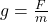## WILL GIVE BRAINLIEST Suppose an elephant has a mass of 1,800 kg and a person has a mass of 75 kg. If the strength of the gravitationa

Question

WILL GIVE BRAINLIEST
Suppose an elephant has a mass of 1,800 kg and a person has a mass of 75 kg. If the strength of the gravitational force on the person was 735 N, what would be the gravitational force on the elephant? (Assume both the person and elephant are on Earth’s surface.)

in progress 0
5 months 2021-08-12T14:47:07+00:00 1 Answers 160 views 0

Making g the subject thenand substituting F with 735 N and m with 75 for the case of a person, g is equivalent to 735/75=9.8